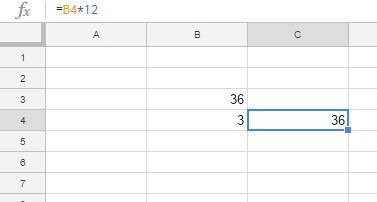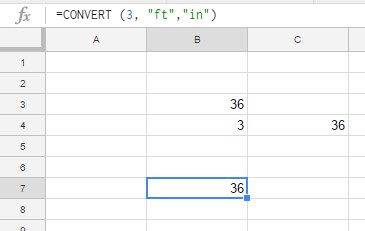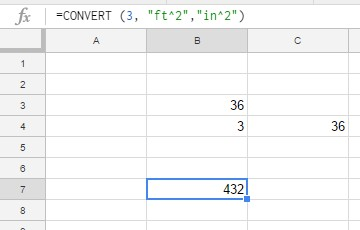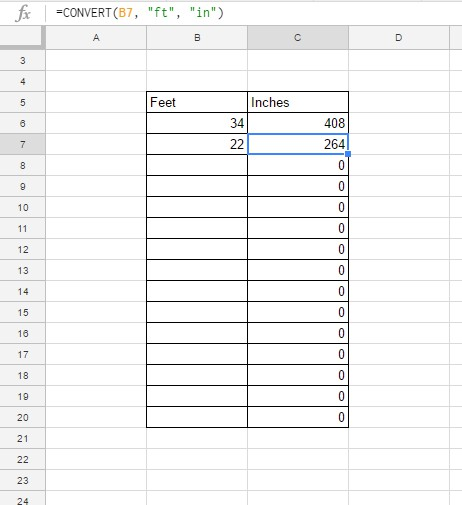# How To Convert Feet to Inches in Google Sheets

If you don’t have Excel on a desktop or laptop, you can set up spreadsheets with Google Sheets instead. This is a web app that shares many Excel functions. CONVERT is one of the handy Sheets functions that converts various units for distance, time, energy, volume, area, speed and more besides. This is how Google Sheets users can convert feet to inches in spreadsheets.## Convert Feet to Inches Without a Function

You can convert feet to inches in Google Sheets without a function by entering a formula in the fx bar. There are 12 inches in a foot, so you can convert feet to inches by multiplying any value by 12. Alternatively, convert inches to feet by dividing the number of inches by 12.

Open a blank Google Sheets spreadsheet, and then select cell B3. Click in the fx bar, enter ‘3*12’ and press the Return key. B3 will return a value of 36. Three feet amounts to 36 inches.Alternatively, you can enter the foot value in a spreadsheet cell first. Enter ‘3’ in cell B4, and then select to add the function to cell C4. Enter ‘=B4*12’ in the function bar. Now cell C4 includes the value 36 as shown in the snapshot directly below.To convert inches to feet, you’ll need to divide the units. Select to add the inches to feet formula to cell B5. Then type ‘=55/12’ in the function bar. Cell B5 will return 4.58 as the total number of inches in 55 feet.

## Convert Feet to Inches with CONVERT

Although it’s not essential for most unit conversion, it’s probably better to convert feet to inches with the CONVERT function. The syntax for this function is: CONVERT(value, start_unit, end_unit). Value is the number to convert, and the start and end units in the function are the conversion units.

For an example, select B7 in your Google Sheets spreadsheet. Then enter ‘=CONVERT (3, “ft”,”in”)’ in the fx bar. Cell B7 returns the value 36 inches when you press Enter. In that function “ft” (feet) is the start unit and “in” (inch) the end unit. To convert inches to feet, enter the function as ‘=CONVERT (3, “in”,”ft”)’ in the fx bar. To include a cell reference, you would enter the value in B7; and then input the function as ‘=CONVERT (B7, “ft”,”in”)’ in another cell.The function can also include area units. It enables you to convert feet values to square inches. Replace the “ft” and “in” units in the brackets with “ft^2” and “in^2” instead. For example, edit the previous CONVERT function entered in B7 of your Google Sheets spreadsheet by replacing the feet and inch units with “ft^2” and “in^2” in the brackets. Then the function will be =CONVERT (3, “ft^2″,”in^2”), and it will return the value 432 square inches as shown directly below.You can also add sheet references to the CONVERT function. This enables you to add the function to an entirely different sheet in the spreadsheet than the one that includes the number to convert to inches. For an example, enter ‘7’ in cell B9 of Sheet 1. Then click the + Add Sheet button to add Sheet2 to the spreadsheet as shown below.Select B3 in Sheet2 to include the conversion function. Enter ‘=CONVERT(Sheet1!B9, “ft”, “in”)’ in the function bar. B3 will return the value 84, which is otherwise the total of seven feet * 12 inches. To include a sheet reference, add the sheet’s title followed by an exclamation mark in the function’s brackets first.## Set up a Foot to Inches Conversion Table

Now you can set up a spreadsheet table for converting feet to inches. Open a blank Google Sheets spreadsheet, and starting from row 5 drag your cursor over a group of cells in column B and C by holding the left mouse button. You must select an equal number of cells in both columns. Press the Borders button, and select the option shown in the snapshot directly below. Your spreadsheet table should be comparable to the one below.Enter ‘Feet’ in B5 at the top of your table. Enter ‘Inches’ in cell C5 as the header for column C. Input the function ‘=CONVERT(B6, “ft”, “in”)’ in cell C6. You can copy that function to all the table’s cells by left-clicking the bottom right corner of C6. Hold the left button and drag the blue box over all the cells you need to copy the function to. All the cells in column C of the table will convert feet values in column B to inches.That table will certainly come in handy for converting lots of feet values to inches. You can set up a variety of conversion tables in Google Sheets for feet, inches and other units of measure much the same. To convert feet and inches in Excel spreadsheets, check out this Tech Junkie article.

## 2 thoughts on “How To Convert Feet to Inches in Google Sheets”Allan Marcus says:
=concatenate(TO_TEXT(floor(B1/12)),”‘ “,to_text(mod(B1,12)),””””)

where the value in inches is in cell B1. Of course you can move this around at will.Eddie says:
Hi, unfortunately this does not address how to convert partial feet values, like 8′ 9″ into pure inches. I have a data sheet with 48 entries of those values and would like to save time calculating each value!
Otherwise good tutorial.Bri says:
I have the same scenario as Eddie.. is this possible?